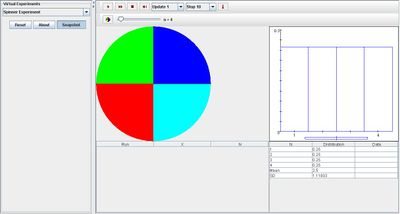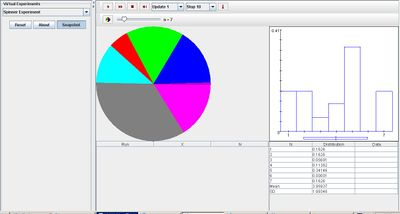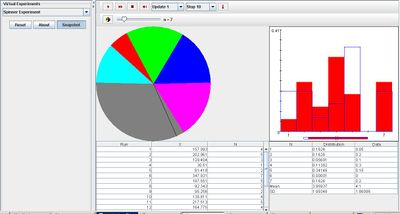# SOCR EduMaterials Activities SpinnerExperiment

(diff) ← Older revision | Latest revision (diff) | Newer revision → (diff)

## Description

The experiment consists spinning a spinner and recording the outcome (the sectors are numbered). The number of sectors in the spinner can be varied with the scroll-bar and the probability of each sector (which is proportional to the area of the sector) can be set with the probability dialog. The probability density function and moments of the random variable (the spinner score) are shown in blue in the distribution graph and recorded numerically in the distribution table. When the experiment runs, the empirical density and moments are shown in red in the distribution graph and recorded in the distribution table. In single step mode, the spinner pointer actually spins, and a sound is played whose tone depends on the outcome.

## Goal

The main focus of the Spinner Experiment is to provide an accessible simulation that demonstrates the properties of proportional probability. By using this applet, users should be able to develop a generalization of events with equal probability, or specified probability of distinct outcomes.

## Experiment

Go to the SOCR Experiment [SOCR Experiment] and select the Spinner Experiment from the drop-down list of experiments on the top left. The image below shows the initial view of this experiment:When pressing the play button, one trial will be executed and recorded in the distribution table below. The fast forward button symbolizes the nth number of trials to be executed each time. The stop button ceases any activity and is helpful when the experimenter chooses “continuous,” indicating an infinite number of events. The fourth button will reset the entire experiment, deleting all previous information and data collected. The “update” scroll indicates nth number of trials (1, 10, 100, or 1000) performed when selecting the fast forward button and the “stop” scroll indicates the maximum number of trials in the experiment.

Increasing parameter n increases the number of sections to n. The probability dialog box can be modified by the user and the sections will change accordingly. The image shown below demonstrates this property as the proportions of each section has been changed:After many trials, the empirical density graph converges to the distribution graph as demonstrated below:## Applications

The Spinner Experiment is a simple application that can be used in many different types of situations such as:

Suppose marketers want to demonstrate the probabilities of customers randomly selecting specified products under their production. After calculating the probability of each product being represented in the overall population, they begin using this applet to illustrate experiments of customers randomly selecting their product.

Suppose statisticians want to illustrate the probability of selecting a specific race within the population of admitted students in college. By using this applet, they can easily present their findings.